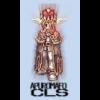Tuts 4 You

# [KeyGenMe] T.P.o.D.T's KeyGenMe #1## Recommended PostsHi,

For the group T.P.o.D.T I created a KeyGenMe... It has no public encryption and is just based on my own fantasy...

I hope you guys can solve it...

Solution = Keygen + Source + Tutorial (really nice for newbies learning)...

Mr. eXoDia // T.P.o.D.T 2011

mr.exodia.tpodt@gmail.com
/>http://www.tpodt.net16.net

Edited by Mr. eXoDiaWeird I attached it too but apparently it failedMr. eXoDia

PS Already solved it?Edited by Mr. eXoDiaI made a quick keygen in masm, but I ripped your whole algo. I'm too lazy not to rip it.Easier and quicker that way.where is the fun ripping the code ?

here is my quick try, code can be optimized.

tnx for the keygenme.

`var  i, j, x, Len: Integer;  Name: string;  Name2: string;  Buffer: array of Char;  tmp1, tmp2, tmp3:Integer;begin  Name:= Edt1.Text;  for i := 1 to 12 do    Name2 := Name2 + Name;  Len := Length(Name2);  i:= 1 ;  j:= 0 ;  x:= 6;  SetLength(Buffer,13);  repeat    begin      Buffer[j] := c h r(ord(Name2[i]));      inc(j);      Buffer[j] := c h r(ord(Name2[Len]));      Dec(Len,2);      Inc(i,2);      inc(j);      Dec(x)    end;  until x = 0 ;  Name := '';  i := 0;  j := 12;  repeat    tmp1 := ord(Buffer[j]);    tmp2 := ord(buffer[i]);    tmp3 := tmp1 + tmp2;    Name := Name + Format('%X%X%X',[tmp1,tmp2,tmp3]);    inc(i);    Dec(j);  until i= 12;  Len:= Length(Name);  Name := Copy(Name,2,Len);  Len:= Length(Name);  Name2 := '';  for i := 1 to Len do    begin      tmp1 := StrToInt('\$'+Name[i]);      if tmp1 <> 9 then        inc(tmp1);      Name2 := Name2 + IntToHex(tmp1,0);      if i mod 10 = 0 then        Name2 := Name2 + '-';    end;  Name2 := copy(Name2,1,Length(name2)-1);  Edt2.Text := Name2;end;`@sama:

True that. I might make another one in BC++ if I have time, just to practice C++.

And you already posted Delphi source...@sama: Maybe spoiler the code block...? (I don't know if there are spoilers on this site btw)

@saduff: yes it would be nice if you code it for 'real'...

Mr. eXoDia